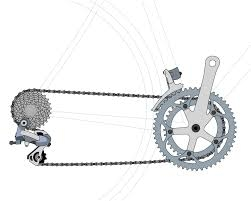# Four bicycles

In the department store, they sell bicycles for families with children. Women's bicycles are 10% more expensive than men's, and children's bikes are a third cheaper than women's. The family paid a total of CZK 31,030 for two children's, one man's, and one woman's bike. How many crowns did each round cost?

d =  9570 Kc
p =  8700 Kc
c =  6380 Kc

### Step-by-step explanation:Did you find an error or inaccuracy? Feel free to write us. Thank you!

Math student
Let x be the price of a man’s bike, y be the price of a woman’s bike, and z be the price of a child’s bike. Then, we can write the following equations:

y = 1.1x (women’s bikes are 10% more expensive than men’s)

z = 0.67y (children’s bikes are a third cheaper than women’s)

2z + x + y = 31030 (the family paid a total of CZK 31,030 for two children’s, one man’s, and one woman’s bike)

To solve this system, we can use the method of elimination12 or the Wolfram|Alpha calculator1. The solution is:

x = 9000 (the price of a man’s bike)

y = 9900 (the price of a woman’s bike)

z = 6615 (the price of a child’s bike)

Tomas2001
2/3 is not 0.67 .... so

Tips for related online calculators
Need help calculating sum, simplifying, or multiplying fractions? Try our fraction calculator.